Related Articles

# Time Series Analysis using Facebook Prophet

• Last Updated : 30 Jun, 2020

Prophet is an open-source tool from Facebook used for forecasting time series data which helps businesses understand and possibly predict the market. It is based on a decomposable additive model where non-linear trends are fit with seasonality, it also takes into account the effects of holidays. Before we head right into coding, let’s learn certain terms that are required to understand this.

Trend:
The trend shows the tendency of the data to increase or decrease over a long period of time and it filters out the seasonal variations.

Seasonality:
Seasonality is the variations that occur over a short period of time and is not prominent enough to be called a “trend”.

Understanding the Prophet Model
The general idea of the model is similar to a generalized additive model. The “Prophet Equation” fits, as mentioned above, trend, seasonality and holidays. This is given by,

y(t) = g(t) + s(t) + h(t) + e(t)

where,

• g(t) refers to trend (changes over a long period of time)
• s(t) refers to seasonality (periodic or short term changes)
• h(t) refers to effects of holidays to the forecast
•  e(t) refers to the unconditional changes that is specific to a business or a person or a circumstance. It is also called the error term.
•  y(t) is the forecast.

This seems easy enough, so why do we need a tool like Prophet to help us with forecasting?
We need it because, although the basic decomposable additive model looks simple, the calculation of the terms within is hugely mathematical and, if you do not know what you are doing, may lead to making wrong forecasts which might have severe repercussions in the real world. So to automate this process, we are going to use Prophet.

However, to understand the math behind this process and how Prophet actually works, let’s see how it forecasts the data.
Prophet provides us with two models(however, newer models can be written or extended according to specific requirements). One is the logistic growth model and the other one is piece-wise linear model. By default, Prophet uses piece-wise linear model, but it can be changed by specifying the model. Choosing a model is delicate as it is dependent on a variety of factors such as company size, growth rate, business model etc., If the data to be forecasted, has saturating and non-linear data(grows non-linearly and after reaching the saturation point, shows little to no growth or shrink and only exhibits some seasonal changes), then logistic growth model is the best option. Nevertheless, if the data shows linear properties and had a growth or shrink trends in the past then, piece-wise linear model is a better choice.

The logistic growth model is fit using the following statistical equation,

(1)where,

• C is the carry capacity
• k is the growth rate
• m is an offset parameter

Piece-wise linear model is fit using the following statistical equations,

(2)where c is the trend change point(it defines the change in the trend). ? is trend parameter and can be tuned as per requirement for forecasting.

Now let’s use this knowledge with a real example.  Consider the air passengers data set(please open the link below and save the .csv file)
https://raw.githubusercontent.com/rahulhegde99/Time-Series-Analysis-and-Forecasting-of-Air-Passengers/master/airpassengers.csv

The above data-set contains the number of air passengers in USA from January 1949 to December 1960. The frequency of the data is 1 month. Now let’s try and build a model that is going to forecast the number of passengers for the next five years using time series analysis.

### Installations

Install Pandas for data manipulation and for the dataframe data structure.

pip install pandas


Install Prophet for time series analysis and forecasting.

pip install fbprophet


Note: If you don’t want to install the modules locally, use Jupyter Notebooks or Google Colab.
Implementation:
Code: Import all the modules required

 import pandas as pdfrom fbprophet import Prophetfrom fbprophet.plot import add_changepoints_to_plot

 data = pd.read_csv('https://raw.githubusercontent.com/rahulhegde99/Time-Series-Analysis-and-Forecasting-of-Air-Passengers/master/airpassengers.csv')data.head()

Output: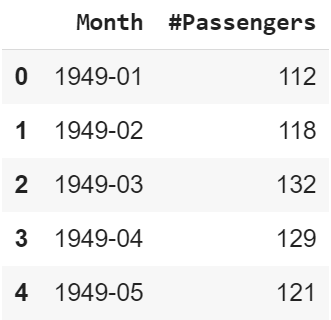Facebook Prophet predicts data only when it is in a certain format. The dataframe with the data should have column saved as ds for time series data and y for the data to be forecasted. Here, the time series is the column Month and the data to be forecasted is the column #Passengers. So let’s make a new dataframe with new column names and the same data. Also, ds should be in a datetime format.

Code:

 df = pd.DataFrame()df['ds'] = pd.to_datetime(data['Month'])df['y'] = data['#Passengers']df.head()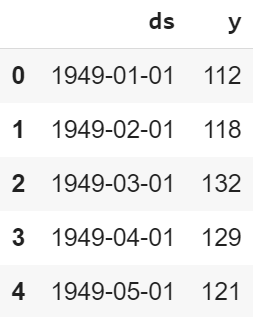Code: Initialize a model and fit our dataframe df to it.

 m = Prophet()m.fit(df)

We want our model to predict for the next 5 years, that is, till 1965. The frequency of our data is 1 month and thus for 5 years, it is 12*5=60 months. So we need to add 60 to more rows of monthly data to a dataframe.
Code:

 future = m.make_future_dataframe(periods=12 * 5, freq='M')

Now in the future dataframe we have just ds values and we should predict the y values.
Code:

 forecast = m.predict(future)forecast[['ds', 'yhat', 'yhat_lower', 'yhat_upper', 'trend', 'trend_lower', 'trend_upper']].tail()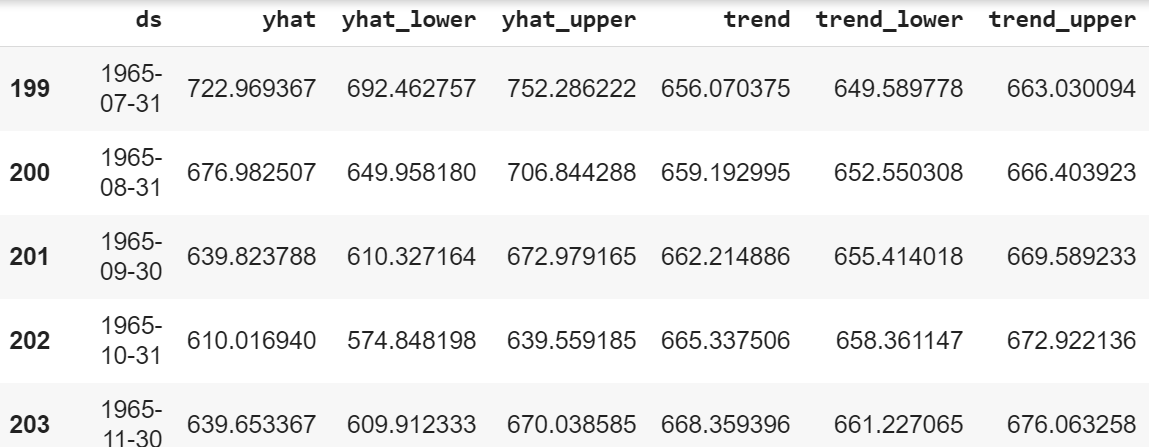In the table ds, as we know, is the time series data. yhat is the prediction, yhat_lower and yhat_upper are the uncertainty levels(it basically means the prediction and actual values can vary within the bounds of the uncertainty levels). Next up we have trend which shows the long term growth, shrink or stagnancy of the data, trend_lower and trend_upper are the uncertainty levels.

Code: Plot the forecasted data.

 fig1 = m.plot(forecast)

The below image shows the basic prediction. The light blue is the uncertainty level(yhat_upper and yhat_lower), the dark blue is the prediction(yhat) and the black dots are the original data. We can see that the predicted data is very close to the actual data. In the last five years, there is no “actual” data, but looking at the performance of our model in years where data is available it is safe to say that the predictions are close to accurate.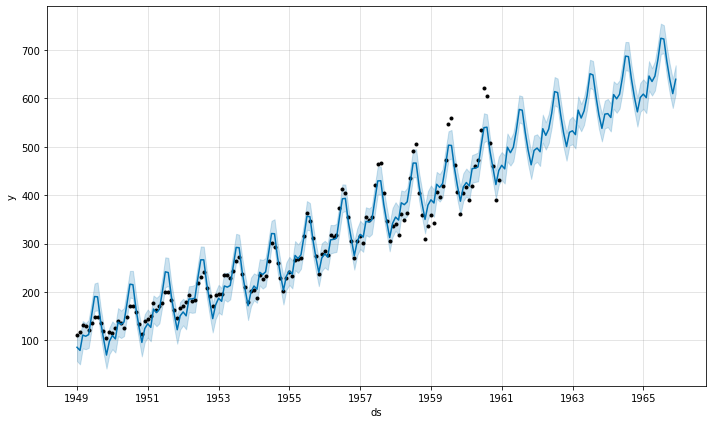fig2 = m.plot_components(forecast)

The below images shows the trends and seasonality(in a year) of the time series data. We can see there is an increasing trend, meaning the number of air passengers has increased over time. If we look at the seasonality graph, we can see that June and July is the time with most passengers at a given year.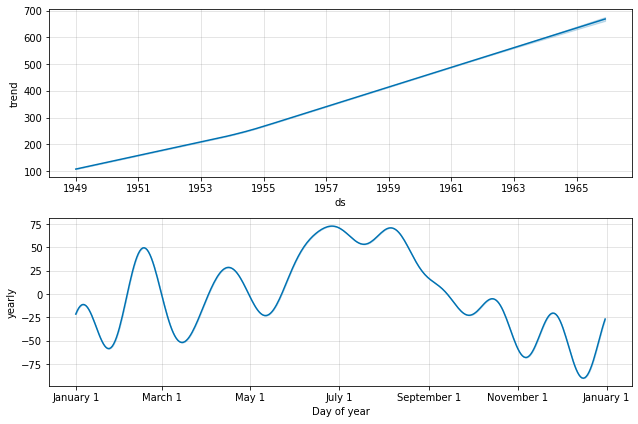fig = m.plot(forecast)a = add_changepoints_to_plot(fig.gca(), m, forecast)

Add changepoints to indicate the time in rapid trend growths. The dotted red lines show the time when there was a rapid change in the trend of the passengers.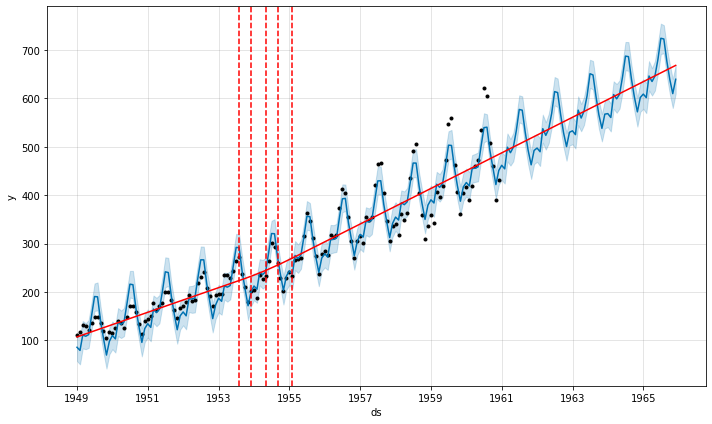Footnotes:
Thus, we have seen how we can design a prediction model using Facebook Prophet with only a few lines of code which would have been very difficult to implement using traditional machine learning algorithms and mathematical and statistical concepts alone.

Attention geek! Strengthen your foundations with the Python Programming Foundation Course and learn the basics.

To begin with, your interview preparations Enhance your Data Structures concepts with the Python DS Course. And to begin with your Machine Learning Journey, join the Machine Learning – Basic Level Course

My Personal Notes arrow_drop_up